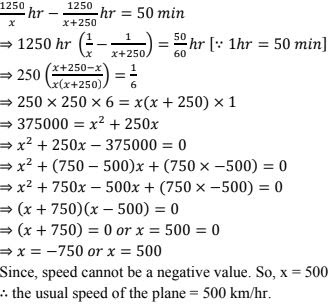# RD Sharma Solutions Chapter 8 Quadratic Equations Exercise 8.8 Class 10 Maths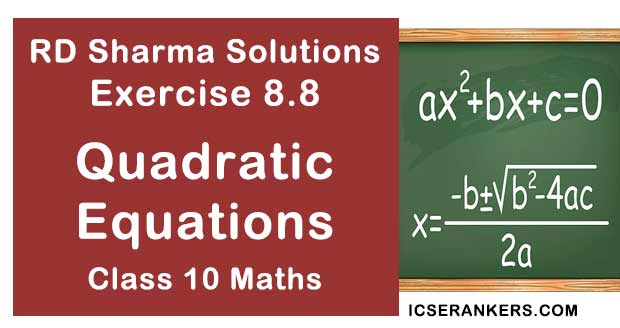Chapter Name RD Sharma Chapter 8 Quadratic Equations Book Name RD Sharma Mathematics for Class 10 Other Exercises Exercise 8.1Exercise 8.2Exercise 8.3Exercise 8.4Exercise 8.5Exercise 8.6Exercise 8.7Exercise 8.9Exercise 8.10Exercise 8.11Exercise 8.12Exercise 8.13 Related Study NCERT Solutions for Class 10 Maths

### Exercise 8.8 Solutions

1. The speed of a boat in still water is 8 km/hr. It can go 15 km upstream and 22 km downstream in 5 hours. Find the speed of the stream.

Solution

Let the speed of the stream be x km/hr
Given that,
Speed of the boat in still water = 8 km/hr
Now,
Speed of the boat in upstream = (8 - x) km/hr
And speed of the boat in downstream = (8 + x) km/hr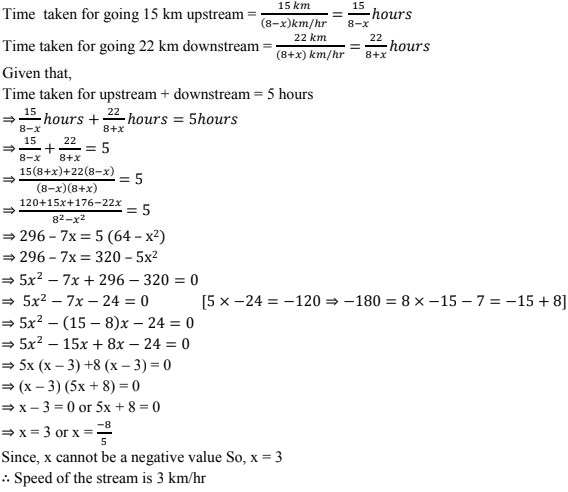2. A passenger train takes 3 hours less for a journey of 360 km if its speed is increased by 10 km/hr from its usual speed. What is the usual speed?
Solution
Let the usual speed be x km/hr.
Distance covered in the journey = 360 km
Now,
Time taken by the train with the usual speed = 360 km/x km/hr = 360/x hr
given that if speed is increased by 10 km/hr, the same train takes 3 hours less.
⇒ Speed of the train = (x + 10) km/hr and time taken by the train after increasing the speed
= 360 km/(x + 10)km/hr = 360/(x + 10) hr

3. A fast train takes one hour less than a slow train for a journey of 200 km. If the speed of the slow train is 10 km/hr less than that of the fast train, find the speed of the two trains.
Solution
Let the speed of the slow train be x km/hr.
given that speed of the slow train is 10  km/hr less than that of fast train
⇒ Speed of the fast train  = (x + 10) km/hr
Total distance covered in the journey = 200 km
Time taken by fast train = 200 km/(x + 10)km/hr = 200/(x + 10) hr and
Time taken by slow train = 200 km/x km/hr = 200/x hr
Given that faster train takes 1 hour less than that of slow train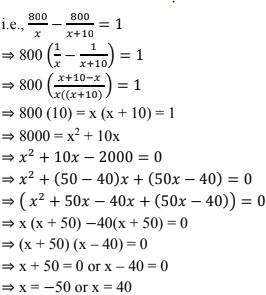Clearly x cannot be a negative volume since it is speed. So, x = 40
∴ Speed of slow train is 40 km/hr
Now,
Speed of fast train = (x + 10) km/hr = (40 + 10)km/hr  = 50 km/hr

4. A passenger train takes one hour less for a journey of 150 km if its speed is increased by 5 km/hr from its usual speed. Find the usual speed of the train.
Solution
Let the usual speed of the train be x km/hr
Distance covered in the journey  = 150 km
Time taken by the train with usual speed = 150 km/x km/hr = 150/x hr
given that, if the speed is increased by 5km/hr from to usual speed, the train takes one hour less for the same journey.
⇒ Speed of the train = (x + 5)km/hr
Now, time taken by the train after increasing the speed = 150 km/(x+ 5) km/hr = 150/(x + 5) hr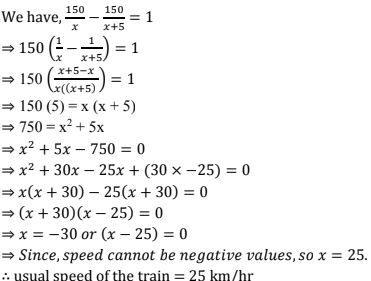5. The time taken by a person to cover 150 km was 2.5  hrs more than the time taken in the return journey. If he returned at a speed of 10 km/hr more than the speed  =o  going, what was the speed per hour in each direction?
Solution
Let the going speed of the person be x km/hr
Given that, the return speed is 10 km/hr  more than the going speed
Return speed of the person = (x + 10) km/hr
Total distance covered = 150 km.
Time taken for going = 150 km/x km/hr = 150/x hr
Time taken for returning  = 150 km/(x + 10) km/hr = 150/(x + 10) hr
Given that, time taken for going in 2.5 hours more than the time for returning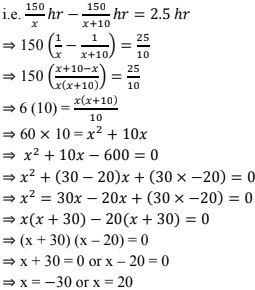Since, speed cannot be negative. So x = 20
∴ speed of the person when going = 20 km/hr
Now, speed of the person when returning = (x + 10) km/hr
= (20 + 10) km/hr
= 30 km/hr

6. A plane left 40 minutes late due to bad weather and in order to reach its destination, 1600 km away in time, it had to increase its speed by 400 km/hr from its usual speed. Find the usual speed of the plane.
Solution
Let the usual speed of the plane be x km/hr
Total distance travelled = 1600 km
⇒ Time taken by the plane with usual speed = 1600 km/x km/hr  = 1600/x hr
Given that, if speed is increased by 400 km/hr, the plane takes 40 minutes less than that of the usual time. Speed of the plane after increasing  = (x + 400) km/hr
⇒ Time taken by the plane with increasing speed = 1600 km/(x + 400) km/hr = 1600/(x + 400) hr
Now,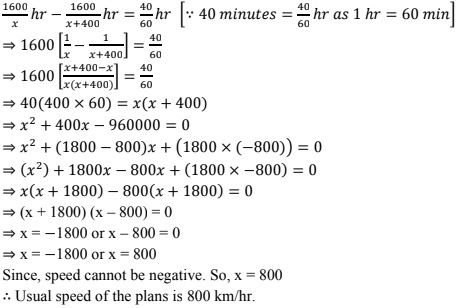7. An aeroplane takes 1 hour less for a journey of 1200 km if its speed is increased by 100 km/hr from its usual speed. Find its usual speed.
Solution
Let the usual speed of the plane be x km/hr .
Distance covered in the journey = 1800 km
⇒ Time taken by the plane with usual speed = 1200 km/ x km/hr  = 1200/x hr
Now, speed is increased by 100 km/hr and the time taken is 1 hour less for the same journey.
⇒ Speed of the plane after increased = (x + 100) km/hr and Time taken by plane with increased speed = 1800 km/(x + 100) km/hr  = 1800/(x + 100) hr
Now, we have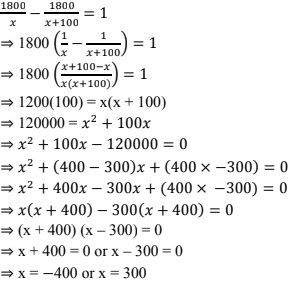8. A passenger train takes 2 hours less for a journey of 300 km if its speed is increased by 5 km/hr from its usual speed. Find the usual speed of the train.
Solution
Let the usual speed of the train be x km/hr
Distance covered in the journey = 300 km
Time taken by the train with usual speed = 300 km /x km/hr  = 300/x hr
Now,
If the speed is increased by 5 km/hr, the train takes 2 hours less for the same journey.
Speed of the train after increasing = (x + 5) km/hr
And time taken by the train after increasing the speed = 300 km/(x + 5)km/hr  = 300/(x + 5) hr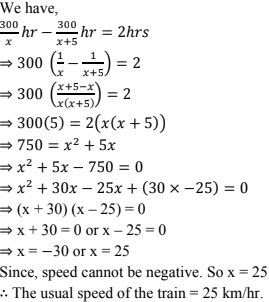9. A train covers a distance of 90 km at a uniform speed. Had the speed been 15 km/hour more, it would have taken 30 minutes less for the journey. Find the original speed of the train.
Solution
Let the original speed of the train be x km/hr
Distance covered  = 90 km.
Time taken by the train with original speed = 90km/x km/hr = 90/x hr
Now, if the speed of the train is increased by 15 km/hr, the train takes 30 minutes less for the same journey
Speed of the train after increasing = (x + 15) km/hr and the time taken by the train after increasing the speed = 90km/(x + 15) km/hr  = 90/(x + 15) hr
Now,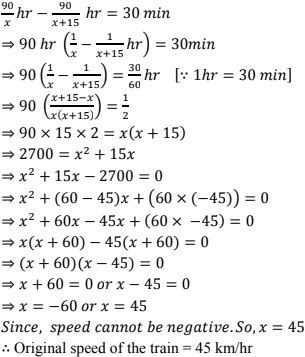10. A train travels 360 km at a uniform speed. If the speed had been 5 km/hr more, it would have taken 1 hour less for the same journey. Find the speed of the train.
Solution
Let the speed of the train be x km/hr
Distance covered by the train = 360 km
⇒ Time taken by the train with initial speed = 360 km/x km/hr = 360/x hr
Now, if the speed is 5 km/hr more, the train takes 1 hour less for the same journey.
Speed of the train after increasing the speed = (x + 5) km/hr
And time taken by the the train with increased speed = 360km/(x + 5) km/hr  = 360/(x + 5) hr
Now,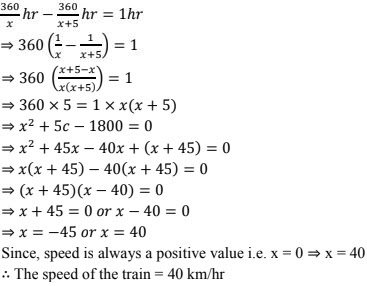11. An express train takes 1 hour less than a passenger train to travel 132 km between Mysore and Bangalore(without taking into consideration the time they stop at intermediate stations). If the average speed of the express train is 11 km/hr more than that of the passenger train, find the average speed of the two trains.
Solution
Let the speed of the passenger train be x km/hr
Given that the average speed of the express train is 11 km/hr more than that of passenger train.
Average speed of express train = (x + 1) km/hr
Now,
Time taken by the passenger train = 138 km/x km/hr  = 13/x hr
And time taken by the express train = 132 km/(x + 11) km/hr  = 138/(x + 11) hr
Given that, express train takes 1 hour less than that of passenger train to reach the destiny.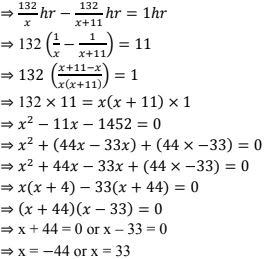Since, speed cannot be in negative values.
∴ Average speed of the slower train i.e. passenger train = 33 km/h
And average speed of express train = (x + 11) km/hr  = (33 + 11) km/hr  = 44  km/hr .

12. An aeroplane left 50 minutes later than its scheduled time, and in order to reach the destination, 1250 km away, in time, it had to increase its speed by 250 km/hr from its usual speed. Find its usual speed.
Solution
Let the usual speed of the plane be x km/hr
Distance covered by the plane = 1250 km
Time taken by the plane with usual speed = 1250 km/x km/hr  = 1250 hr
To cover the delay of 50 minutes, the speed of the plane in increased by 250 km/hr
Now,
Speed of the plane after increasing = (x + 250) km/hr and
Time taken by the plane with increased speed = 1250 km/(x + 250) km/hr = 1250/(x + 250) hr
From the data we have,